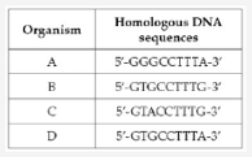# Problem: Which of the following statements is true of the parsimony method for reconstructing phylogeny?a. The preferred phylogenetic tree under the parsimony method is the tree with the fewest branches. b. Parsimony assumes that once a character changes, it never reverses to the previous state. c. The preferred phylogenetic tree under the parsimony method is the tree that requires the smallest number of evolutionary changes to explain the data. d. Parsimony assumes that evolutionary convergences do not occur between biological lineages. Refer to the table below. The table summarizes base sequences of homologous regions of DNA in four different organisms. If A represents the outgroup and B, C, and D represent the ingroup under study, how would a phylogenetic tree be constructed to show the evolutionary relationships between B, C, and D? a. B branches off first, followed by D, and then C. b. D branches off first, followed by B, and then C. c. B branches off first, followed by C, and then D. d. C branches off first, followed by D, and then B.

###### Problem Details

Which of the following statements is true of the parsimony method for reconstructing phylogeny?

a. The preferred phylogenetic tree under the parsimony method is the tree with the fewest branches.

b. Parsimony assumes that once a character changes, it never reverses to the previous state.

c. The preferred phylogenetic tree under the parsimony method is the tree that requires the smallest number of evolutionary changes to explain the data.

d. Parsimony assumes that evolutionary convergences do not occur between biological lineages.

Refer to the table below.The table summarizes base sequences of homologous regions of DNA in four different organisms. If A represents the outgroup and B, C, and D represent the ingroup under study, how would a phylogenetic tree be constructed to show the evolutionary relationships between B, C, and D?

a. B branches off first, followed by D, and then C.

b. D branches off first, followed by B, and then C.

c. B branches off first, followed by C, and then D.

d. C branches off first, followed by D, and then B.